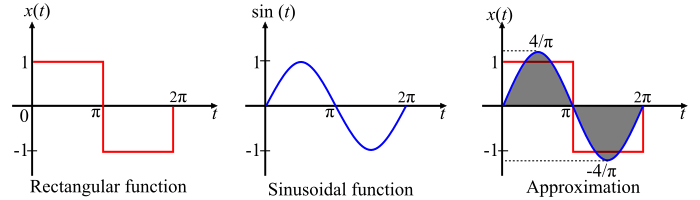# What is Mean Square Error?

The mean square error (MSE) is defined as mean or average of the square of the difference between actual and estimated values.

Mathematically, the mean square error is,

$$\mathrm{\varepsilon =\frac{1}{t_{2}-t_{1}}\int_{t_{1}}^{t_{2}}\left [ x(t) -\sum_{r=1}^{n}C_{r}g_{r}(t)\right ]^{2}dt}$$

$$\mathrm{\varepsilon =\frac{1}{t_{2}-t_{1}}\left [ \int_{t_{1}}^{t_{2}}x^{2}(t)dt+\sum_{r=1}^{n}C_{r}^{2}\int_{t_{1}}^{t_{2}}g_{r}^{2}(t)dt-2\sum_{r=1}^{n}C_{r}\int_{t_{1}}^{t_{2}}x(t)g_{r}(t)dt\right ]\; ...(1)}$$

$$\mathrm{\therefore C_{r}=\frac{\int_{t_{1}}^{t_{2}}x(t)g_{r}(t)dt}{\int_{t_{1}}^{t_{2}}g_{r}^{2}(t)dt}=\frac{1}{K_{r}}\int_{t_{1}}^{t_{2}}x(t)g_{r}(t)dt\; \; ...(2)}$$

$$\mathrm{\therefore \int_{t_{1}}^{t_{2}}x(t)g_{r}(t)dt=C_{r}\int_{t_{1}}^{t_{2}}g_{r}^{2}(t)dt=C_{r}K_{r}\; \; ...(3)}$$

Using equations (1) and (3), we have,

$$\mathrm{\varepsilon =\frac{1}{t_{2}-t_{1}}\left [\int_{t_{1}}^{t_{2}} x^{2}(t)dt +\sum_{r=1}^{n}C^{2}_{r}K_{r}-2\sum_{r=1}^{n}C^{2}_{r}K_{r}\right ]}$$

$$\mathrm{\Rightarrow \varepsilon =\frac{1}{t_{2}-t_{1}}\left [\int_{t_{1}}^{t_{2}} x^{2}(t)dt -\sum_{r=1}^{n}C^{2}_{r}K_{r}\right ]\; \; ...(4)}$$

$$\mathrm{\Rightarrow \varepsilon =\frac{1}{t_{2}-t_{1}}\left [ \int_{t_{1}}^{t_{2}}x^{2}(t)dt-(C_{1}^{2}K_{1}+C_{2}^{2}K_{2}+\cdot \cdot \cdot +C_{n}^{2}K_{n}) \right ]\; \; \cdot \cdot \cdot (5)}$$

Therefore, the mean square error can be evaluated using eqn. (5).

## Numerical Example

A rectangular function is defined as,

$$\mathrm{x(t)=\left\{\begin{matrix} 1\; \; for\, 0< t< \Pi \ -1\; \; for\, \Pi< t< 2\Pi\ \end{matrix}\right.}$$

The signal x(t) is approximated to a sinusoidal function $\mathrm{x(t)=\frac{4}{\Pi }\sin t}$ sin 𝑡 over the interval [0, 2π]. Evaluate the mean square error in this approximation.

## Solution

The approximation of the rectangular function x(t) by a sinusoidal signal sin 𝑡 is shown in the figure and is given by,

$$\mathrm{x(t)=\frac{4}{\Pi }\sin t}$$The mean square error in this approximation can be evaluated using the formula,

$$\mathrm{\Rightarrow \varepsilon =\frac{1}{t_{2}-t_{1}}\left [\int_{t_{1}}^{t_{2}} x^{2}(t)\: dt-\int_{t_{1}}^{t_{2}} \left ( \frac{4}{\pi }\sin t \right )^{2}\; dt\right] }$$

Here, 𝑡1 = 0 and 𝑡2 = 2𝜋, therefore,

$$\mathrm{\Rightarrow \varepsilon =\frac{1}{2\pi -0}\left [\int_{0}^{2\pi } 1\: dt-(\frac{4}{\pi })^{2}\int_{0}^{2\pi } \sin^{2} t\; dt\right] }$$

$$\mathrm{\Rightarrow \varepsilon =\frac{1}{2\pi}\left [\int_{0}^{2\pi }\: dt-\left ( \frac{4}{\pi } \right )^{2}\int_{0}^{2\pi } \left ( \frac{1-cos2t}{2} \right )\; dt\right] }$$

$$\mathrm{\Rightarrow \varepsilon =\frac{1}{2\pi}\left [\int_{0}^{2\pi }\: dt-\left ( \frac{16}{\pi^{2} } \right )\int_{0}^{2\pi } \left ( \frac{1-cos2t}{2} \right )\; dt\right] }$$

$$\mathrm{\Rightarrow \varepsilon =\frac{1}{2\pi}\left [\left [ t \right ]_{0}^{2\pi }-\frac{16}{2\pi ^{2}}\left [ t-\frac{sin2t}{2} \right ]_{0}^{2\pi }\right] }$$

$$\mathrm{\Rightarrow \varepsilon =\frac{1}{2\pi }\left [ \left ( 2\pi -0 \right )-\frac{8}{\pi ^{2}}\begin{Bmatrix} (2\pi -0)-\left ( \frac{sin4\pi -sin0}{2} \right )\ \end{Bmatrix} \right ]}$$

$$\mathrm{\Rightarrow \varepsilon =\frac{1}{2\pi }\left [ 2\pi -\frac{16}{\pi} \right ]=1-\frac{8}{\pi ^{2}}=0.189}$$

∴ Mean square error, 𝜀 = 0.189 = 18.9%